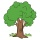Home Communities
IT Knowledge
Inspiration
Languages
EN

# Python - join two lists

0 points
Created by:Mahir-Bright
501

In this article, we would like to show you how to join two lists in Python.

## 1. Using `+` operator

In this example, we use `+` operator to join two lists.

Practical example:

``````list1 = ['A', 'B']
list2 = ['C', 'D']

result = list1 + list2

print(result)  # ['A', 'B', 'C', 'D']``````

Output:

``['A', 'B', 'C', 'D']``

## 2. Using `extend()` method

In this example, we use `extend()` method to add `list2` at the end of `list1`.

Practical example:

``````list1 = ['A', 'B']
list2 = ['C', 'D']

list1.extend(list2)

print(list1)  # ['A', 'B', 'C', 'D']``````

Output:

``['A', 'B', 'C', 'D']``

## 3. Append items one by one

In this example, we use `append()` method to append items from `list2` at the end of `list1` one by one.

Practical example:

``````list1 = ['A', 'B']
list2 = ['C', 'D']

for x in list2:
list1.append(x)

print(list1)  # ['A', 'B', 'C', 'D']``````

Output:

``['A', 'B', 'C', 'D']``

## Alternative titles

Join to our subscribers to be up to date with content, news and offers.